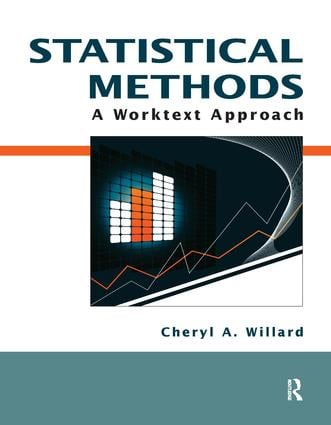Statistical Methods

A Worktext Approach, 1st Edition

Routledge

242 pages

Purchasing Options:\$ = USD
Paperback: 9781884585906
pub: 2010-01-01
SAVE ~\$22.00
\$110.00
\$88.00
x
eBook (VitalSource) : 9781315265803
pub: 2016-10-04
from \$55.00

FREE Standard Shipping!

Description

• Statistical Methods: A Worktext Approach uses a student-friendly, conversational tone to explain core statistical concepts in a way that students can readily understand.

• After concepts and skills are introduced and demonstrated, students are given a hands-on opportunity to work with the concepts and practice their newly acquired skills with Your Turn exercises.

• Each chapter begins with a careful explanation of the statistical concepts relevant to that chapter. Following the explanation of concepts are examples that illustrate their applications.

• Boxes containing helpful pointers for students are included in the chapters. These are often mnemonics for remembering concepts and precautions warning against common mistakes.

• Statistical terms are in boldfaced type and italicized. They are defined in the text and also appear in the glossary in Appendix A.

• An extensive instructor’s guide provides answers to the exercises.

Chapter 1: Introduction to Statistics

Chapter 2: Organizing Data Using Tables and Graphs

Chapter 3: Measures of Central Tendency

Chapter 4: Measures of Variability

Chapter 5: z-Scores and Other Standard Scores

Chapter 6: Probability and the Normal Distribution

Chapter 7: Sampling Distribution of Means

Chapter 8: Hypothesis Testing

Chapter 9: One-Sample t Test

Chapter 10: Two-Sample t Test: Independent Samples Design

Chapter 11: Two-Sample t Test: Related Samples Design

Chapter 12: Confidence Interval versus Point Estimation

Chapter 13: One-Way Analysis of Variance

Chapter 14: Correlation and Regression

Chapter 15: Chi-Square

Appendix A: Glossary of Statistical Terms

Appendix B: Glossary of Statistical Formulas

Table 1: Table of the Normal Distribution Curve

Table 2: Critical Values of the t-Distribution

Table 3: Critical Values of F for the .05 Level

Table 4: Critical Values of F for the .01 Level